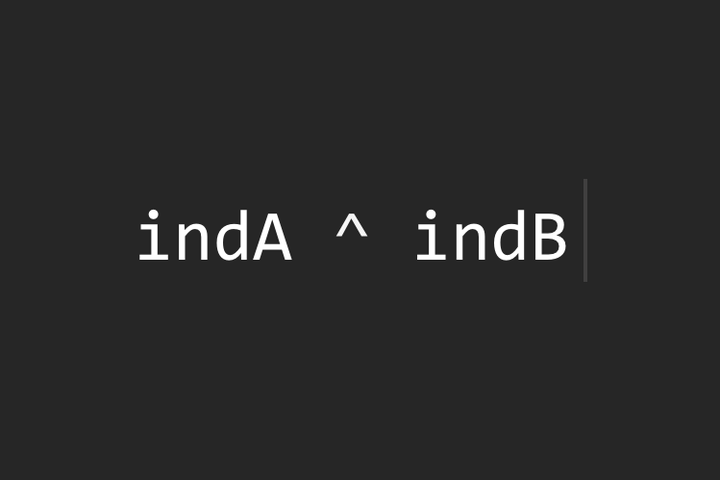Pandas中的对象简介
Python 数据科学手册(抢先版)

```import numpy as np
import pandas as pd
```

Pandas Series

Series是一个包含被索引的数据的一维的数组。它可以由如下的列表或者数组创建而成：

```data = pd.Series([0.25, 0.5, 0.75, 1.0])
data```

`data.values`

`data.index`

`data`
`data[1:3]`

```data = pd.Series([0.25, 0.5, 0.75, 1.0],
index=['a', 'b', 'c', 'd'])
data
```

`data['b']`

```data = pd.Series([0.25, 0.5, 0.75, 1.0],
index=[2, 5, 3, 7])
data
```
`data`

```population_dict = {'California': 38332521,
'Texas': 26448193,
'New York': 19651127,
'Florida': 19552860,
'Illinois': 12882135}
population = pd.Series(population_dict)
population
```

`population['California']`

`population['California':'Illinois']`

>>> pd.Series(data, index=index)

`pd.Series([2, 4, 6])`

data也可以是一个标量，该标量将会被用以填充所有给定的Index:

`pd.Series(5, index=[100, 200, 300])`

data可以是一个字典，其中默认的index是排序后的字典键:

`pd.Series({2:'a', 1:'b', 3:'c'})`

```pd.Series({2:'a', 1:'b', 3:'c'}, index=[3, 2])
```

Pandas DataFrame

```area_dict = {'California': 423967, 'Texas': 695662, 'New York': 141297, 'Florida': 170312, 'Illinois': 149995}
area = pd.Series(area_dict)
area
```

```states = pd.DataFrame({'population': population,
'area': area})
states
```

```states.index
```

```states.columns
```

```states['area']
```

```pd.DataFrame(population, columns=['population'])
```

```data = [{'a': i, 'b': 2 * i}
for i in range(3)]
pd.DataFrame(data)
```

```pd.DataFrame([{'a': 1, 'b': 2}, {'b': 3, 'c': 4}])
```

```pd.DataFrame({'population': population,
'area': area})
```

```pd.DataFrame(np.random.rand(3, 2),
columns=['foo', 'bar'],
index=['a', 'b', 'c'])
```

```A = np.zeros(3, dtype=[('A', 'i8'), ('B', 'f8')])
A
```
```pd.DataFrame(A)
```

Pandas Index

```ind = pd.Index([2, 3, 5, 7, 11])
ind
```

Index中很多的操作与数组类似。例如，我们可以用标准的Python下标表示法来获取值或者切片：

```ind
```
```ind[::2]
```

Index对象也有许多在NumPy数组中我们熟知的属性：

```print(ind.size, ind.shape, ind.ndim, ind.dtype)
```

Index对象和NumPy数组的一个不同点是，Index是不可变的，也即通过一般的途径是不能修改的。

```ind = 0
```

Pandas对象被设计成便于如数据集之间的连接等操作，这些操作都依赖于集合运算的许多方面。回想一下，我们在第X.X节中详细介绍过，Python有一个内置的集合对象。Index对象遵循了很多这一内置集合对象的习惯用法，使得并、交、差以及其他组合运算能以熟悉的方法来计算：

```indA = pd.Index([1, 3, 5, 7, 9])
indB = pd.Index([2, 3, 5, 7, 11])
```
```indA &amp; indB  # intersection
```
```indA | indB  # union
```
```indA - indB  # difference
```
```indA ^ indB  # symmetric difference
```Pandas table